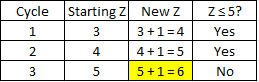## Complex Code

The following pseudocode snippet describes a segment of a computer program. What value of Z is printed?
SET A = 10
SET B = 5
SET C = 1
SET X = 2
SET Y = 4
SET Z = 3
While Z ≤ 5
A = A/B + C
B = A*C - 5000
K = Z^5 - 10
C = K + A + B
X = X*Y*Z + A + B + C
Y = A*B*C + X + Y + Z
Z = Z + 1
End While
Print Z

Hint
The code is running a loop. $$Z$$ increases incrementally every time the code cycles through.
Hint 2
Factor in the exit criteria for the loop to end. You’ll only need to track the $$Z$$ values for each cycle.
The code is running a loop. $$Z$$ increases incrementally every time the code cycles through. Because the exit criteria is based on $$Z$$ , we’ll only need to track $$Z$$ for every cycle since it’s independent of the other variables. The other variables and their associated equations simply exist to cause confusion and take up time. Running through the script logically:The loop is exited once $$Z = 6$$ . Therefore, the value of $$Z$$ that is printed is 6.
6